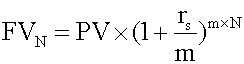Tags
Question

When compounding periods are other than annual the formula is:Tags
Question

When compounding periods are other than annual the formula is:

?

Tags
Question

When compounding periods are other than annual the formula is:If you want to change selection, open document below and click on "Move attachment"

subject 3. The Future Value and Present Value of a Single Cash Flow
. When you calculate these amounts, make sure that periodic interest rates correspond to the number of compounding periods in the year. For example, if time periods are quoted in quarters, quarterly interest rates should be used. <span>When compounding periods are other than annual r s = the quoted annual interest rate m = the number of compounding periods per year N = the number of years. Example 2 An analyst invests \$5 million in a 5-year certificate of deposit (CD) at a local financial institution. The CD promises to pay 7% per year, compounded semi-a

#### Summary

status measured difficulty not learned 37% [default] 0

No repetitions